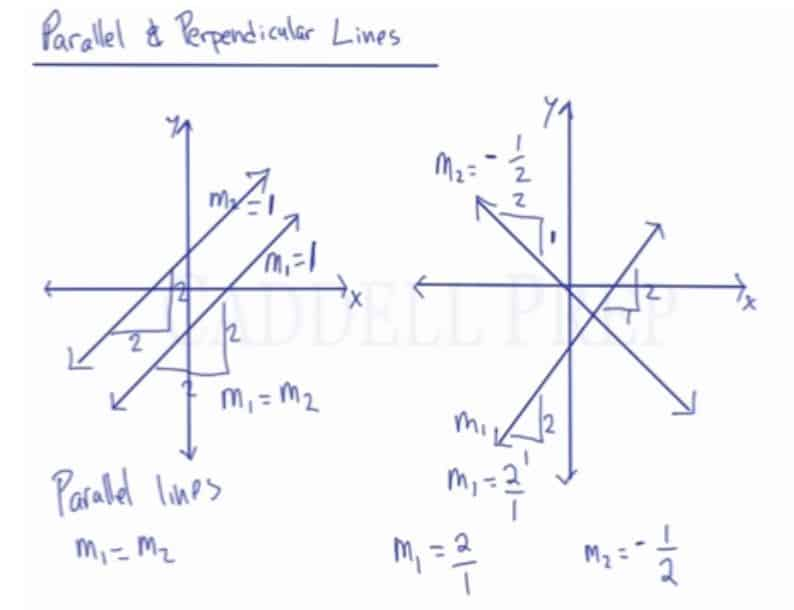Learn about the relationship between parallel and perpendicular lines. After you finish this lesson, view all of our Algebra 1 lessons and practice problems.

Parallel lines have the same slope but different y-intercepts. Ex:$y= 2x+7$ and$y=2x-3$ are parallel.

Perpendicular lines have slopes that are the negative reciprocals of each other and may or may not have the same y-intercept. Ex:$y= 4x+3$ and$y=-\dfrac{1}{4}x+4$ are perpendicular.## Examples of Parallel And Perpendicular Lines

### Example 1$y=\dfrac{2}{3}x+5$$y=\dfrac{2}{3}x-1$Both have the same slope which is$\dfrac{2}{3}$

Therefore, the lines are parallel to each other.

### Example 2$y=-4x+10$$y=\dfrac{x}{4}+\dfrac{1}{4}$The slopes are negative reciprocals of each other$m_1=-4$$m_2=\dfrac{1}{4}$

Therefore, the lines are perpendicular.

## Video-Lesson Transcript

Let’s go over parallel and perpendicular lines.

We have two graphs each with a line.

The one on the left side has a positive slope. The line is going upwards from left to right.

If we draw a line parallel to it, it would look something like this.

If the first line is going to run over$2$ and rise at$2$, then the second line will show the same exact rate.

So, if the slope of the first line is$m_1$ and the slope of the second line is$m_2$, we can say that$m_1 = m_2$.

So if$m_1 = 1$ then we can say that$m_2 = 1$.

Now, let’s look at the second graph.

Let’s say that this line is over$1$ and up$2$, the slope here is$m_1 = \dfrac{2}{1}$.

Now if we draw a line perpendicular with this, it will look something like this.

The thing with a perpendicular line is that it goes the other way. If the first line is going up, then the perpendicular line goes down. If one line has a positive slope, then the other line has a negative slope.

Now if the first line over$1$ and up$2$, the perpendicular line is going over$2$ and down$1$.

So the slope of the second line is$m_2 = -\dfrac{1}{2}$

Let’s look at these closely.$m_1 = \dfrac{2}{1}$$m_2 = -\dfrac{1}{2}$

These two slopes are the negative reciprocal of each other.

Therefore, in parallel lines the slopes are equal$m_1 = m_2$.

While the perpendicular line is the negative reciprocal of each other.